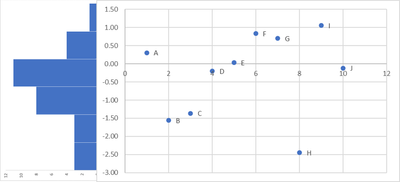## Histogram and Scatterplot Sharing an Axis

I would like to create a graphic in which a histogram that shows the frequencies for one variable (Ability in the data example, Have) shares an axis with a scatterplot of two other variables (X = Item and Y = Difficulty in the data example). That is, the values of Ability and Difficulty would all reference a common axis as shown in the Want figure below. Ideally, the scatterplot points would be labeled with another variable (Label in the data example). Any idea whether and, if so, how this can be done in SAS?

Have:Want:4 REPLIES 4

## Re: Histogram and Scatterplot Sharing an Axis

Yes, it can definitely be done. GTL is likely what you'd need though. I would probably create each graph type separate first and then combine them.

If you use SGPLOT to create each graph individually and then use the TMPLOUT option you'll get the GTL code.
Then you have to figure out how to set up the layout of them.

I highly recommend searching on lexjansen.com for GTL papers for examples.

FYI - If you post images we'd have to type out your data to work with it since we cannot copy/paste.

## Re: Histogram and Scatterplot Sharing an Axis

You might look at the code here: https://support.sas.com/kb/35/172.html

This shows a scatter plot with histograms of both the x and y variable. So removing the x variable bits for the histogram may do what you are looking for.

Warning: it does not show the count for the histogram bar lengths.Ksharp
Super User

## Re: Histogram and Scatterplot Sharing an AxisKsharp
Super User

## Re: Histogram and Scatterplot Sharing an Axis

Discussion stats
• 4 replies
• 392 views
• 2 likes
• 4 in conversation